Download Presentation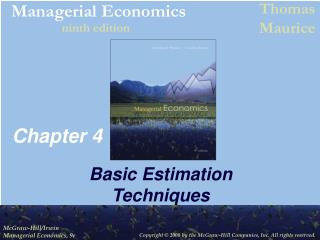Chapter 4

# Chapter 4 - PowerPoint PPT Presentation

Chapter 4. Basic Estimation Techniques. •. Slope parameter ( b ) gives the change in Y associated with a one-unit change in X ,. Simple Linear Regression. Simple linear regression model relates dependent variable Y to one independent (or explanatory) variable X.I am the owner, or an agent authorized to act on behalf of the owner, of the copyrighted work described.
Download Presentation## Chapter 4

An Image/Link below is provided (as is) to download presentation

Download Policy: Content on the Website is provided to you AS IS for your information and personal use and may not be sold / licensed / shared on other websites without getting consent from its author.While downloading, if for some reason you are not able to download a presentation, the publisher may have deleted the file from their server.

- - - - - - - - - - - - - - - - - - - - - - - - - - E N D - - - - - - - - - - - - - - - - - - - - - - - - - -
Presentation Transcript### Chapter 4

Basic Estimation Techniques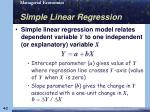• Slope parameter (b) gives the change in Y associated with a one-unit change in X,
Simple Linear Regression
• Simple linear regression model relates dependent variable Y to one independent (or explanatory) variable X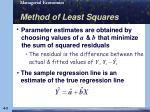Method of Least Squares
• The sample regression line is an estimate of the true regression line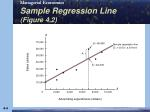ei

Sample Regression Line (Figure 4.2)

S

70,000

Sales (dollars)

60,000

50,000

40,000

30,000

20,000

10,000

A

10,000

4,000

2,000

8,000

6,000

0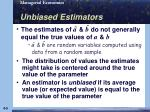Unbiased Estimators
• The distribution of values the estimates might take is centered around the true value of the parameter
• An estimator is unbiased if its average value (or expected value) is equal to the true value of the parameter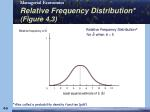Relative Frequency Distribution* (Figure 4.3)

1

4

2

3

8

9

10

5

6

7

0

1

*Also called a probability density function (pdf)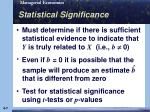Statistical Significance
• Must determine if there is sufficient statistical evidence to indicate that Y is truly related to X (i.e., b 0)
• Test for statistical significance using t-tests orp-values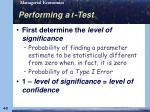Performing a t-Test
• First determine the level of significance
• Probability of finding a parameter estimate to be statistically different from zero when, in fact, it is zero
• Probability of a Type I Error
• 1 – level of significance = level of confidence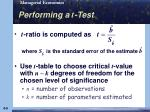Performing a t-Test
• Use t-table to choose critical t-value with n – k degrees of freedom for the chosen level of significance
• n = number of observations
• k = number of parameters estimated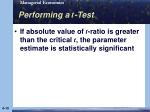Performing a t-Test
• If absolute value of t-ratio is greater than the critical t, the parameter estimate is statistically significantUsing p-Values
• Treat as statistically significant only those parameter estimates with p-values smaller than the maximum acceptable significance level
• p-value gives exact level of significance
• Also the probability of finding significance when none existsCoefficient of Determination
• R2 measures the percentage of total variation in the dependent variable that is explained by the regression equation
• Ranges from 0 to 1
• High R2indicates Y and X are highly correlatedF-Test
• Used to test for significance of overall regression equation
• Compare F-statistic to critical F-value from F-table
• Two degrees of freedom, n – k & k – 1
• Level of significance
• If F-statistic exceeds the critical F, the regression equation overall is statistically significantMultiple Regression
• Uses more than one explanatory variable
• Coefficient for each explanatory variable measures the change in the dependent variable associated with a one-unit change in that explanatory variableis U-shapedor

U

-shaped